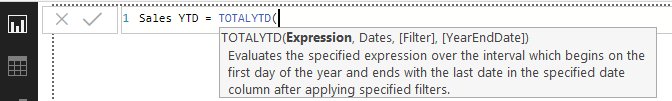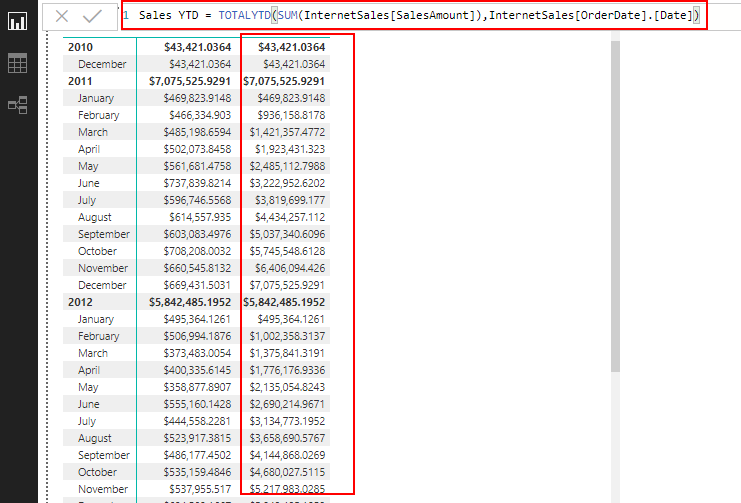# Power BI – TOTALYTD DAX Time Intelligence FunctionWhat is TOTALYTD function?

Let us consider a scenario where you are working on a report in Power BI where you need to calculate the sales for the year-to-date. TOTALYTD is a DAX Time Intelligence Function which will help you in this scenario. TOTALYTD evaluates the year-to-date value of the expression in the current context. Please keep in mind that there are several other ways too for calculating year-to-date using DAX.

Syntax

```TOTALYTD(<expression>,<dates>[,<filter>][,<year_end_date>])
```
• <expression> : An expression that returns a scalar value like SUM, COUNT etc.
• <dates> : A column that contains dates.
• <filter> : (optional) An expression that specifies a filter to apply to the current context.
• <year_end_date> : (optional) A literal string with a date that defines the year-end date. The default is December 31.

TOTALYTD returns a scalar value that represents the expression evaluated for the current year-to-date dates.

The dates argument can be any of the following:

• A reference to a date/time column,
• A table expression that returns a single column of date/time values,
• A Boolean expression that defines a single-column table of date/time values. But using Boolean expression have below constraints
• The expression cannot reference a measure.
• The expression cannot use a nested CALCULATE function.
• The expression cannot use any function that scans a table or returns a table, including aggregation functions.
• However, a Boolean expression can use any function that looks up a single value, or that calculate a scalar value.

Using TOTALYTD in Power BI

Now let us check how to use TOTALYTD in Power BI. Consider below example of a report on Sales for each MonthLet us try to add a Year to Date measure to this report. We will use the TOTALYTD DAX function to achieve this.We need to calculate the sum of SalesAmount for the year-to-date. So we will do a SUM as an expression over the Date field.

```Sales YTD = TOTALYTD(SUM(InternetSales[SalesAmount]),InternetSales[OrderDate].[Date])
```We can use the optional Filter parameter to filter the results. For example, if we need to calculate year-to-date sales for a particular Territory

```Sales YTD = TOTALYTD(SUM(InternetSales[SalesAmount]),InternetSales[OrderDate].[Date],InternetSales[SalesTerritoryKey]=4)
```1.supriyana says: